e-ISSN: 2319-9849

All submissions of the EM system will be redirected to Online Manuscript Submission System. Authors are requested to submit articles directly to Online Manuscript Submission System of respective journal.

# Conductance Studies on Complex Formation between Nano Nickel Sulphate and Eosin Y in MeOH Solutions at Different Temperatures

Esam A Gomaa1*, Elsayed M Abou Elleef2, Amr A Ibrahim1 and Amir M Abbas1

1Chemistry Department, Faculty of Science, Mansoura University, 35516-Mansoura, Egypt

2Basic Science Department, Delta Higher Institute for Engineering & Technology, 35681- Dalkha, Mansoura, Egypt

*Corresponding Author:
Esam A Gomaa
Chemistry Department, Faculty of Science
Mansoura University, 35516-Mansoura, Egypt

Received date: 13/05/2014; Revised date: 22/06/2014; Accepted date: 24/06/2014

Visit for more related articles at Research & Reviews: Journal of Chemistry

## Abstract

This article summarizes different aspects of the complexes of the the nano NiSO4 with ligand (Eosin Y) as studied condutometrically in pure MeOH solvent at 293.15, 298.15, 303.15 and 308.15K by applying the condutometric method. Consequently, we will study the effect of solvent properties, (MeOH) on stoichiometry, the selectivity between ligand and ions in various systems and thermodynamic parameters of complexation. On drawing the relation between molar conductance and the ratio of metal to ligand concentrations, different lines are obtained indicating the formation of 1:2, and 1:1 (M:L) stoichiometry complexes. The stability constant of the complexes were obtained from fitting the molar conductivity curves using a computer program. This research focused on the study of thermodynamic complexation reactions between the ligand, Eosin Y, with Ni2+ metal cation in pure MeOH solvent.

#### Keywords

Complexation; formation constant; Gibbs free energy; enthalpy and entropy of complexation.

#### Introduction

The study of the interactions involved in the complexation of different cations with ligand in solvent mixtures is important for a better understanding of the mechanism of biological transport, molecular recognition, and other analytical applications.

Recently, there has been much research on complex formation. There are a number of physico-chemical techniques that can be used in the study of these complexation reactions, for example, spectrophotometry, polarography, NMR spectrometry, calorimetry, potentiometry and conductometry . Nevertheless of all these techniques, conductometric techniques are the most useful for studying this complexation of complexes. This is because conductometric techniques are highly sensitive and inexpensive, with a simple design of Experimental arrangement for such investigations . Therefore, it offers more benefits than the other methods.

Transition metal ions have a strong role in bio-inorganic chemistry and redox enzyme systems and may provide the basis of models for active sites of biological systems . Eosin Y is considered as a reversible inhibitor of NKA (Na,K-AT Pase, sodium pump) which is competitive with ATP in the absence of K+ ions. Its fluorescence behavior is very sensitive to conformation changes of this enzyme . It was described that the fluorescence of Eosin Y in the presence of NKA is enhanced by Mg2+ , which is an important cofactor for the enzyme activity.

#### Material and methods

All chemicals used were of the highest purity available and were purchased from Merck.

The ligand

Molecular Structure:Preparation of nano nickel sulphate

The nano nickel sulphate was prepared by shaking nickel sulphate in ball-mill apparatus of the type Retsch MM2000 swing mill for a period of one hour. The mill has 10 cm3 stainless steel tube. Two stainless steel balls of 12 mm diameter were used .Ball milling was performed at 20225 Hz at room temperature.

Conductometric titration

In a typical experiment, 5 mL of the Eosin Y solution (1.0 x 10-4 M) was placed in the titration cell, thermostated at the preset temperature and the conductance of the solution was measured after the solution reached thermal equilibrium. Then, a known amount of the NiSO4.6H2O solution (1.0 x 10-3 M) was added in a stepwise manner using a calibrated micropipette. The conductance of the solution was measured after each addition until the desired cation-to-ligand mole ratio was achieved. The specific conductance values were recorded using conductivity bridge HANNA, H1 8819N with a cell constant equal to 1. The conductometer was conducted with a thermostat of the type the Kottermann 4130 ultra thermostat. The temperature was adjusted at 293.15, 298.15, 303.15 and 308.15 K.

#### Results and Discussion

TEM Images

The TEM images of nano nickel sulphate sample in distilled water were measured using electron microscope model Joel 2010 and shown in Fig . (1). The images show almost deformed spherical particle shapes and broken dishes with an average size of 19 and 45 nm, respectively. It was clear from the electron diffraction image that the nano NiSO4 is in crystalline form , with mean value of r1 340 mm (radius of the first ring) and r2 ( mean radius of the second ring) equal 484 mm. The ratio of r2 to r1 equal 1.424. The molar conductance (Λm) values were calculated using equation (1):(1)

Figure 1: TEM images for nano nickel sulphate , (d) is electron diffraction image.

Where Ks and Ksolv are the specific conductance of the solution and the solvent, respectively ; Kcell is the cell constant and C is the molar concentration of the NiSO4.6H2O solution. By drawing the relation between molar conductance (/\m) and the molar ratio of metal to ligand [M]/[L] concentrations different lines are obtained with sharp breaks indicating the formation of 2:1, 1:1 and 1:2 [M : L] stoichiometric complexes, Fig. (2-5).

Figure 2: The relation between molar conductance ( Λm ) and the [M]/[L] molar ratio of nano NiSO4.6H2O to Eosin Y in pure methanol solvent at 293.15 K.

Figure 3: The relation between molar conductance ( Λm ) and the [M]/[L] molar ratio of nano NiSO4.6H2O to Eosin Y in pure methanol solvent at 298.15 K.

Figure 4: The relation between molar conductance ( Λm ) and the [M]/[L] molar ratio of nano NiSO4.6H2O to Eosin Y in pure methanol solvent at 303.15 K.

Figure 5: The relation between molar conductance ( Λm ) and the [M]/[L] molar ratio of nano NiSO4.6H2O to Eosin Y in pure methanol solvent at 308.15 K.

Figure 6: The relation between log Kf for (2:1), (1:1) and (1:2) stoichiometric complexes between nano NiSO4.6H2O and Eosin Y against 1/T.

As is obvious from Fig. (2-5), in all cases studied, addition of the NiSO4.6H2O solution to the ligand (Eosin Y) solutions caused a continuous decrease in the molar conductance of the solutions, indicating the lower mobility of the complexed cations compared to the solvated ones. In all cases, the slope of the molar conductance-mole ratio plots change sharply at the point where the ligand to cation mole ratio is one, indicating the formation of a relatively stable 2:1, 1:1 and 1:2 [M : L] complex between the ligand and the cation solutions used.

The 1:1 binding of the Ni2+ cations with Eosin Y ligand can be expressed by equilibrium: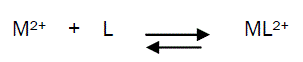and the corresponding formation constants (Kf) for Ni2+-Eosin Y complexes were calculated by using equation:(2)

where [ML2+],[M2+], [L] and f represents the equilibrium molar concentrations of complex, free cation , free ligand and the activity coefficients of the species indicated, respectively.

Under the dilute conditions used, the activity coefficient of the uncharged ligand, fL can be reasonably assumed to as unity [6,7]. The use of Debey-Huckel limiting law of electrolytes  leads to the conclusion that fM2+ & fML2+ , so that the activity coefficients in Eq. 2 cancel out.

Thus, the complex formation constant in terms of the molar conductance can be expressed as [9-11]:(3)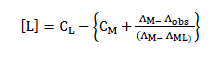(4)

Here, ΛM is the molar conductance of the NiSO4.6H2O solution before addition of the ligand, ΛML the molar conductance of the complex, Λobs the molar conductance of the solution during titration, CL the analytical concentration of the ligand, and CM2+ , the analytical concentration of the NiSO4.6H2O solution. The complex formation constant, Kf, and the molar conductance of the complex, ΛML, were obtained by computer fitting of Eqs. (4) and (5) to the molar conductance- mole ratio data using a nonlinear leastsquares program KINFIT .

The stability constants of the resulting 2:1, 1:1 and 1:2 [M : L] complexes were determined from the computer fitting of Eqs. 3 and 4 to the molar conductance-mole ratio data. A sample computer fit of the mole ratio data is shown in Fig. 1 and all Kf values are summarized in Table 1.

Table 1: The formation constants (log Kf) of formation of of nano NiSO4.6H2O and Eosin Y complexes at different temperatures.

The Gibbs free energies of formation for each stoichiometric complex were calculated by: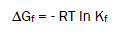(5)

The calculated ΔGf values are presented in tables (2).

Table 2: The Gibbs free energies (ΔGf) of nano NiSO4.6H2O and Eosin Y complexes at different temperatures.

The enthalpy changes of complexation (ΔHf) were calculated from the plots of log Kf against 1/T, (slope = -ΔH/2.303 R) (Fig. 6) using Van’t Hoff eqn . :(6)

Where R is the gas constant and T is the absolute temperature.

Entropy change ΔS were for complexes calculated  by using Gibbs-Helmholtz equation (6)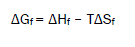(7)

The calculated values of (ΔHf) and (ΔSf) for NiSO4.6H2O-Eosin Y stoichiometric complexes are presented in Table (3).

Table 3: The enthalpies (ΔHf) and entropies (ΔSf) of formation of nan NiSO4.6H2O and Eosin Y complexes at different temperatures.

Enthalpies and entropies of complexation reactions show that, in most cases, the reaction is entropic controlled although, in all cases, a negative value of enthalpic change is also obtained. Comparison of ΔHf values of complexation (Table 1) clearly revealed that the steric hindrance in organic ligand is an important factor in enthalpy changes in the process of complexation reactions. On the other hand, in most cases, the entropy changes during complexation reactions are quite favorable. The entropic change during the complex formation is in fact affected by several factors including the change in flexibility of the reactants in the course of complexation reaction and the differences between the extent of solvation– desolvation of the uncomplexed and complexed species. In the case of the complexation reactions of ligandwith all organic ammonium cations, a large positive ΔSf was obtained. This observation seems to be due to strong solvation of ligand by MeOH ; during the process of complex formation with the organic ammonium ions, these solvent molecules are liberated, causing a favorable entropic change. Interestingly to note, a comparison between the ΔSf, ΔHf and ΔGf values reported in Table 1 reveals that generally a decrease in ΔHf value is accompanied with an increase in ΔSf in such a way that the free energy change ΔGf will remain more or less constant. Such enthalpy-entropy compensation effect was observed earlier in the case of complexation reactions of ligands with cation and inorganic guest species [13-60].

Since the conductance of an ion depends mainly on its mobility, it is quite reasonable to treat the rate process taking place with the change of temperature on the basis of equation(8):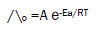(8)

where A is the frequency factor, R is the gas constant and Ea is the Arrhenius activation energy of the transfer process. Consequently, from the plot of log ( ^o) vs. 1/T, the Ea values can be evaluated [15-75] , giving high activation energy value due to solvation behavior.

#### Conclusion

This research focused on the study of thermodynamic complexation reactions between the ligand, Eosin Y, with nano Ni2+ metal cation. The stability constants of the complex formation between ligand and metal cations was investigated by applying the conductometric method at different temperatures. Based on the results, the stability constant for the complexation reaction of Ni2+–Eosin Y shows a decrease with increasing temperatures.

In this thermodynamic study, the negative sign of the ΔGf shows that the ligand is capable of forming stable complexes and that the process will proceeding spontaneously, while the positive sign of the entropy shows that ΔSf is the driving force of the complexation reaction in this complex formation. These facts mean that ΔGf is always negative and ΔSf is always positive.

Replica watches